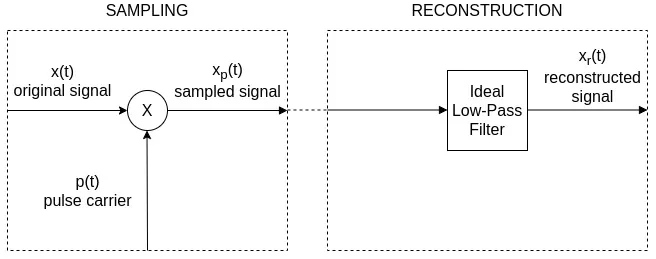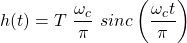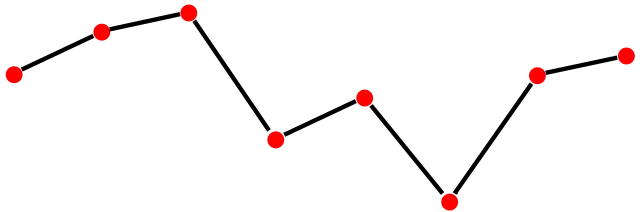# Mathematics - Signals And Systems - Interpolation[Image1]

## Introduction

Hey it's a me again @drifter1!

Today we continue with my mathematics series about Signals and Systems in order to cover Interpolation.

So, without further ado, let's get straight into it!

## Reconstruction ProcedureIn the previous article we based the reconstruction of continuous-time signals that have been sampled on the use of an ideal low-pass filter. This is a natural consequence of interpreting sampling as a procedure in the frequency domain. To get more specific, reconstruction in the time domain is basically a convolution of the impulse train of samples, xp(t), with the impulse response of the low-pass filter, h(t):## Interpolation

The convolution of the samples with the impulse response of the low-pass filter can also be viewed as a superposition of weighted delayed impulse responses, with amplitudes and positions that correspond to the impulses in the impulse train. This superposition represents an interpolation procedure between the samples.

### Band-Limited

When the reconstruction filter is ideal, the interpolation function is a sinc function, and the process is referred to as band-limited interpolation. For such an ideal low-pass filter, with cutoff frqeuency ωc, the impulse response (or interpolation function) is:### Zero-Order Hold

In addition to band-limited interpolation, there are also other commonly used interpolation procedures. For example, there is zero-order hold, which interpolates between sample points by holding each sample value until the next sampling instant. This leads to a staircase-like approximation of the original signal. Zero-order hold corresponds to a convolution with a rectangular pulse interpolation function, with a pulse duration that is equal to the sampling period.[Image 2]

### First-Order Hold (or Linear Interpolation)

Next up there is also linear interpolation, which is also referred to as first-order hold. In this type of interpolation the sample points are interconnected by straight line segments. It basically corresponds to a triangle function with a duration that is exactly twice the sampling period.[Image 3]

## RESOURCES:

### Images

1.2.3.Block diagrams and other visualizations were made using draw.io and GeoGebra

## Previous articles of the series

### Filtering, Sampling, Modulation, Interpolation

• Filtering → Convolution Property, Ideal Filters, Series R-C Circuit and Moving Average Filter Approximations
• Continuous-Time Modulation → Getting into Modulation, AM and FM, Demodulation
• Discrete-Time Modulation → Applications, Carriers, Modulation/Demodulation, Time-Division Multiplexing
• Sampling → Sampling Theorem, Sampling, Reconstruction and Aliasing

## Final words | Next up

And this is actually it for today's post!

Next time we will cover how continuous-time signals are processed as discrete-time signals...

See Ya!Keep on drifting!

H2
H3
H4
3 columns
2 columns
1 column
1 Comment